# GRE Geometry | Triangles

Triangle is one of the basic 2-D shapes in geometry. It consists of three angles, three side and three vertices, e.g.,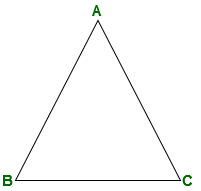On the basis of measurement of sides and angles there different types of triangle listed below:

1. Equilateral triangle:
All edges are equal, all angles are also equal to 60°, e.g.,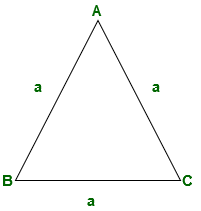2. Isoceles triangle:
Two edges are equal, hrnce two angles are also equal or sides oppsodite to equal sides are equal, e.g.,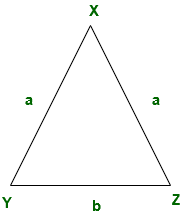3. Scalene triangle:
All edges are different, hence all angles are also different, e.g.,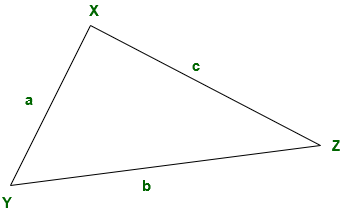4. Right angle triangle:
One angle is 90° and side oppsite to 90° is hypotenuse, e.g.,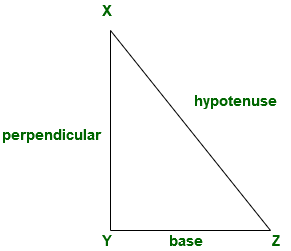5. Obtuse triangle:
One angle is obtuse, e.g.,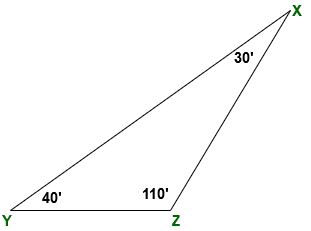6. Acute triangle:
All angles are acute, e.g.,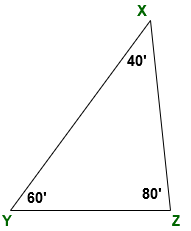Important properties of triangle:

• Sum of angles of triangle is 180°
• Third side of triangle is greater than differce of other two sides and less than sum of other two sides.
• Area of triangle = (1 / 2) * base * height.
• In right angle triangle, hypotenuse2 = base2 + perpendicular2. [Pythagorus theorem]
• Area of equilateral triangle = (√3 / 4)side2

Congurency and similarity in triangles:

1. Congurent triangle:
Two triangles are congurent if shape and size of two triangles is same or two triangle have same angles and same sides, e.g.,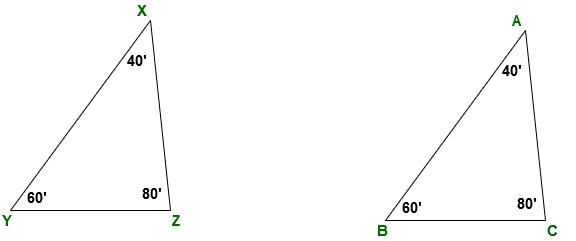Triangle ABC and XYZ are congurent.
or △ABC ≅△XYZ
Rules of congurency:

• (i) SSS (side – side – side) rule:
If all sides of a triangle is equal to all sides of another triangle. Then these two triangles are congurent to each other by SSS rule, e.g.,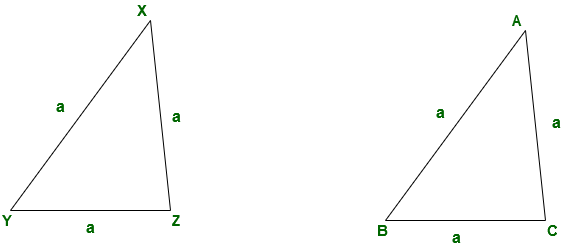• (ii) SAS (side – angle – side) rule:
If two sides of a triangle are equal to two sides in another triangle and angle between them is equal to angle between equal side in another triangle then these two triangles are congurent to each other by SAS rule, e.g.,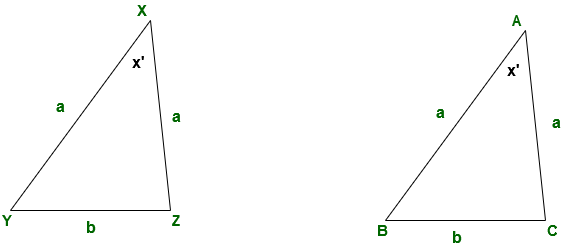• (iii) AAA (angle – angle – angle) rule:If all angles of a triangle are equal to all angles of another triangle the these two triangle are congurent to each other by AAA rule, e.g.,• (iv) ASA (angle – side – angle) rule:
If two angles of a triangle are equal to two angles in another triangle and side between them is equal to side between equal angles in another triangle then these two triangles are congurent to each other by ASA rule, e.g.,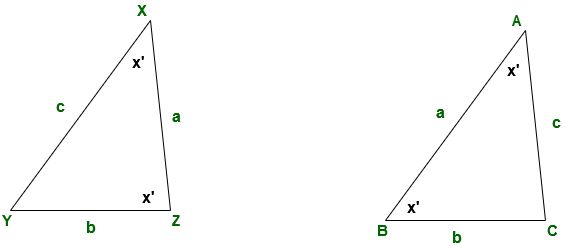• (v) AAS (angle – angle – side) rule:
If two angles and one side of a triangle is equal to two angles and one side in another triangle then these triangles are congurent to each other by AAS rule, e.g.,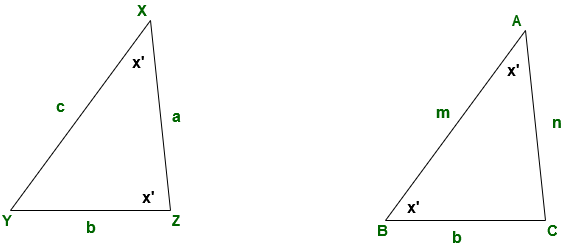2. Similar triangles:
Two triangles are similar if their shape is same but it is not necessary that their size is also same. To prove two triangles are similar scale factor of similarity is used, this factor check the equivalece of ratio of corresponding sides and corresponding angles, e.g.,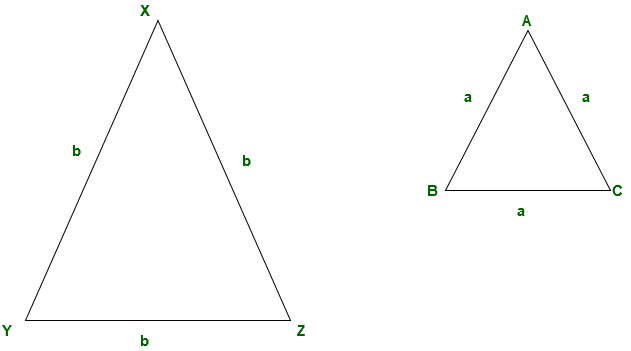`△ABC is similar to △XYZ. `

Since, △ABC is similar to △XYZ we can write scale factor of similarity as:

`XY / AB = XZ / AC = YZ / BC. `

To obtain AB/AC just cross multiply,

```XY / AB = XZ / AC.
AB / AC = XY / XZ.```

My Personal Notes arrow_drop_upCheck out this Author's contributed articles.

If you like GeeksforGeeks and would like to contribute, you can also write an article using contribute.geeksforgeeks.org or mail your article to contribute@geeksforgeeks.org. See your article appearing on the GeeksforGeeks main page and help other Geeks.

Please Improve this article if you find anything incorrect by clicking on the "Improve Article" button below.

Article Tags :

1

Please write to us at contribute@geeksforgeeks.org to report any issue with the above content.